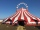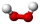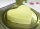# Algebra - math word problems

#### Number of problems found: 2646

• KLMN trapezoidThe KLMN trapezoid has bases KL 40cm and MN 16cm. On the KL base is point P. The segment NP divides the trapezoid into units with the same area. What is the distance of point P from point K?
• CircusOn the circus performance was 150 people. Men were 10 less than women and children 50 more than adults. How many children were in the circus?
• LandRectangular triangular land has area 30 square meters and 12 meters long leg. How many meters of the fence do you need for fencing this land?
• RepairingThree employees earned a total of € 469 for repairing the equipment. They split so that the first got 20% more than the second, and the third 15% more than the second. How many euros did everyone get?
• Math testObelix filled a mathematical test in which he answered 25 questions. For every correct answer, he received 5 points, for each bad answer he had 3 points deducted. Obelix gained 36% of all points in the test. How many questions did he solve correctly?
• The HotelThe Holiday Hotel has the same number of rooms on each floor. Rooms are numbered with natural numbers sequentially from the first floor, no number is omitted, and each room has a different number. Three tourists arrived at the hotel. The first one was in
• Six-digit primesFind all six-digit prime numbers that contain each one of digits 1,2,4,5,7 and 8 just once. How many are they?
• A cylindrical tankA cylindrical tank can hold 44 cubic meters of water. If the radius of the tank is 3.5 meters, how high is the tank?
• Final examThere are 5 learners in a class that has written a final exam Aleta scored 55% vera scored 36% and Sibusiso scored 88% if Thoko scored 71% and the class average was 63%. What was Davids score as a percentage?
• The volume 2The volume of a cube is 27 cubic meters. Find the height of the cube.
• Mini-surveyIn the mini-survey of our class about the popularity of individual subjects, it turned out that 11.1% of pupils like mathematics, 18.5% are enjoying languages, 30.4% of pupils like physical education, and the remaining 12 pupils have several popular subje
• Cube diagonalsCalculate the length of the side and the diagonals of the cube with a volume of 27 cm3.
• CloggingHow much distilled water must the pharmacist add to 30g of a 30% hydrogen peroxide solution to obtain a 3% solution to clogging?
• Rectangle 45The perimeter of a rectangle is 60cm. If the length of the rectangle is 20cm. a)find the width b)find the area.
• Two friendsPeter can do all his work himself in 6 hours. Martin can do the same work himself in 8 hours. Peter worked first and then replaced by Martin. Whole work was done in 6.5 hours. Calculate how long Peter worked before replaced by Martin.
• Diamond diagonalsFind the diamond diagonal's lengths if the area is 156 cm2 and side is 13 cm long.
• Sugar productionFrom 1 ton of beet, 150 kg of sugar is produced. To clean 1 ton of sugar 450 kg of lime is consumed. Calculate how many kgs of lime is consumed when processing 1 ton of sugar beet?
• Three friendsCuba, Matthew and their friend Adam found a brigade during their weekend weekends because they wanted to make a joint trip to the Alps that they planned for the spring break. Cuba enjoyed the skiing trip very much, so he was not lazy to get up and went to
• Area to volumeIf the surface area of a cube is 486, find its volume.
• Two trainsThrough the bridge, long l = 240m, the train passes through the constant speed at time t1 = 21s. A train running along the traffic lights at the edge of the bridge passes the same speed at t2 = 9s. a) What speed v did the train go? b) How long did it ta

Do you have an interesting mathematical word problem that you can't solve it? Submit a math problem, and we can try to solve it.

We will send a solution to your e-mail address. Solved examples are also published here. Please enter the e-mail correctly and check whether you don't have a full mailbox.

Please do not submit problems from current active competitions such as Mathematical Olympiad, correspondence seminars etc...